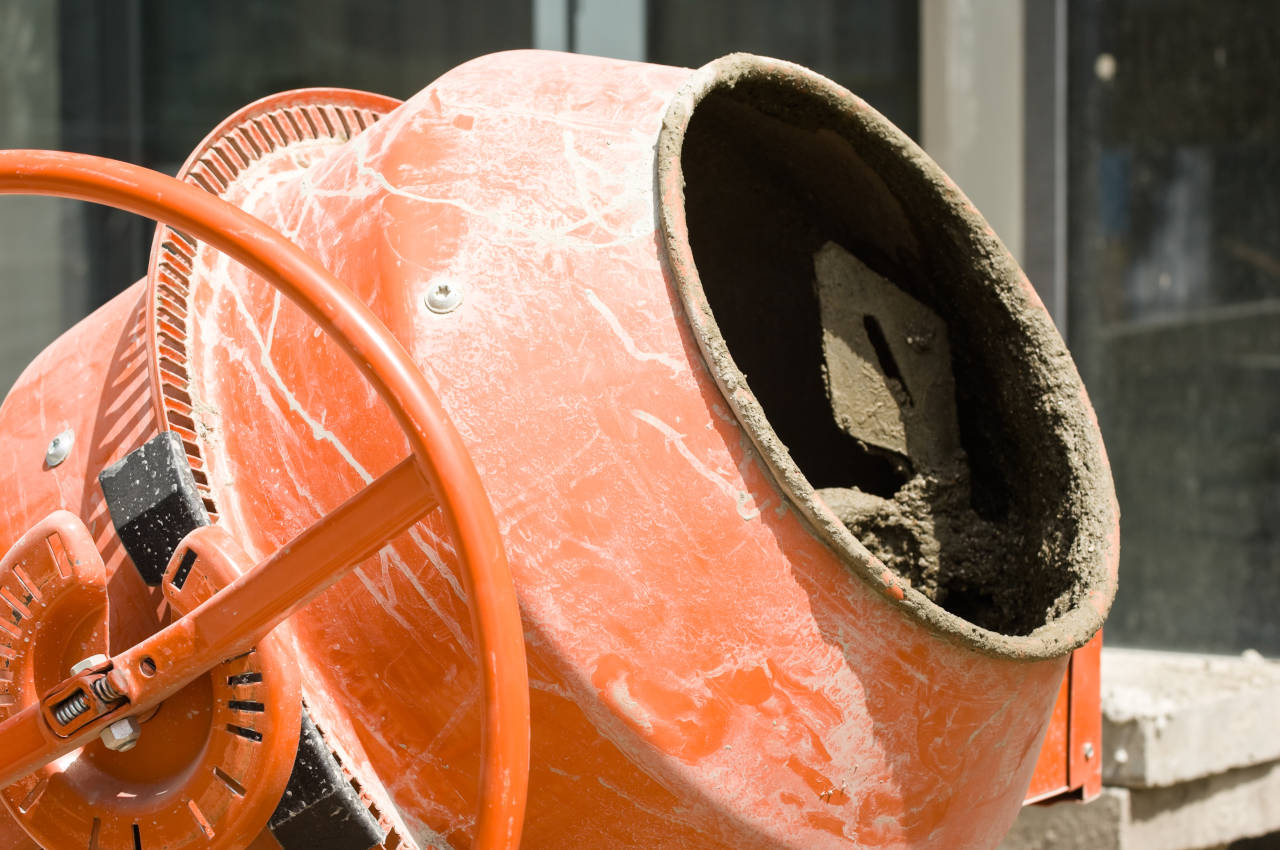# Block Mortar Calculator

Enter the number of bricks or blocks below to calculate how many bags of mortar will be needed, or how much materials will be needed to mix on-site.

Find the number of blocks using our block calculator
Find the number of bricks using our brick calculator

## Material Estimate:

60lb bags

80lb bags

### Custom Mix

Cement

lbs
Hydrated Lime

lbs
Sand

lbs

yds3
*Amounts vary based on labor practices, material & water ratios, and block/brick sizes.## How to Estimate Mortar for Block Projects

Follow three easy steps to estimate the amount of mortar mix needed for your concrete block project.

### Step One: Estimate Square Footage

Estimate the square footage of the wall by measuring the width and height in feet. Then, multiply the width and height to find the square footage. Try calculating square footage using a calculator if you’re not comfortable doing it by hand.

### Step Two: Calculate the Number of Blocks

Next, calculate the number of blocks needed for the project by the number of blocks per square foot. You’ll need roughly 1 1/8 standard blocks per square foot, so multiply the square footage by 1.125 to estimate the total needed. You can also use our block calculator to calculate other block sizes.

### Step Three: Estimate Mortar

The final step will be to find the yield of the mortar you’re using and divide the total number of blocks by the yield. The typical 80-pound bag of mortar will yield 12 blocks so you can calculate by dividing the square footage by 12.

Coverage for mortar mixes and various quantities of blocks.
Block Quantity 60 lb Bags 80 lb Bags
100 blocks 12 bags 9 bags
200 blocks 23 bags 17 bags
300 blocks 34 bags 25 bags

## How to Estimate Mortar for Brick Projects

The same three steps above can be used to estimate mortar for brick projects.

### Step One: Estimate Square Footage

Start by estimating the square footage of the brick wall by multiplying the width by the height together.

### Step Two: Calculate the Number of Bricks

Then, calculate the number of bricks by multiplying the square footage by the number of bricks per square feet. Our brick calculator can help with this. You’ll need roughly 6.9 modular bricks per square foot, so multiply 6.9 times the square footage to find the number of bricks needed.

### Step Three: Estimate Mortar

Finally, estimate the mortar needed by dividing the total number of bricks by the yield of the mix used. On average a mortar mix will yield 36 bricks per 80-pound bag, so divide the total number of bricks by 36 to calculate the bags needed.

Coverage for mortar mixes and various quantities of bricks.
Brick Quantity 60 lb Bags 80 lb Bags
500 bricks 19 bags 14 bags
1,000 bricks 38 bags 28 bags
1,500 bricks 56 bags 42 bags

## Types of Mortar

Whether you’re using a pre-mixed mortar or mixing on-site, it’s important to understand the various types there are and which is appropriate for your project. Cement to sand ratio ranges from 1 part cement to 3 to 4.5 parts sand, depending on the type of mortar being mixed and the compressive strength desired.

Most mixes also require the use of lime in various proportions as a binding agent, to increase the longevity of the finished product, and to increase the workability of the mix.

### Type O

used in above ground applications that are not load-bearing, usually for interior applications, and has a compressive strength of 350psi.

### Type N

used in above ground applications that support light loads, usually for chimneys or brick-work, and has a compressive strength of 750psi.

### Type S

used in below ground applications for load bearing applications, usually for foundations and retaining walls, and has a compressive strength of 1,800psi.

### Type M

used in below ground applications for heavier load bearing applications, usually for foundations to support , and has a compressive strength of 2,500psi.

This table shows the recommended mix proportions and compressive strength for various types of mortar.
Mortar Type Cement : Lime : Sand Ratio Compressive Strength
Type O 1:2:9 350 psi
Type N 1:1:6 750 psi
Type S 2:1:9 1,800 psi
Type M 3:1:12 2,500 psi

Try our other gravel calculator to estimate the amount of sand needed for your mix.